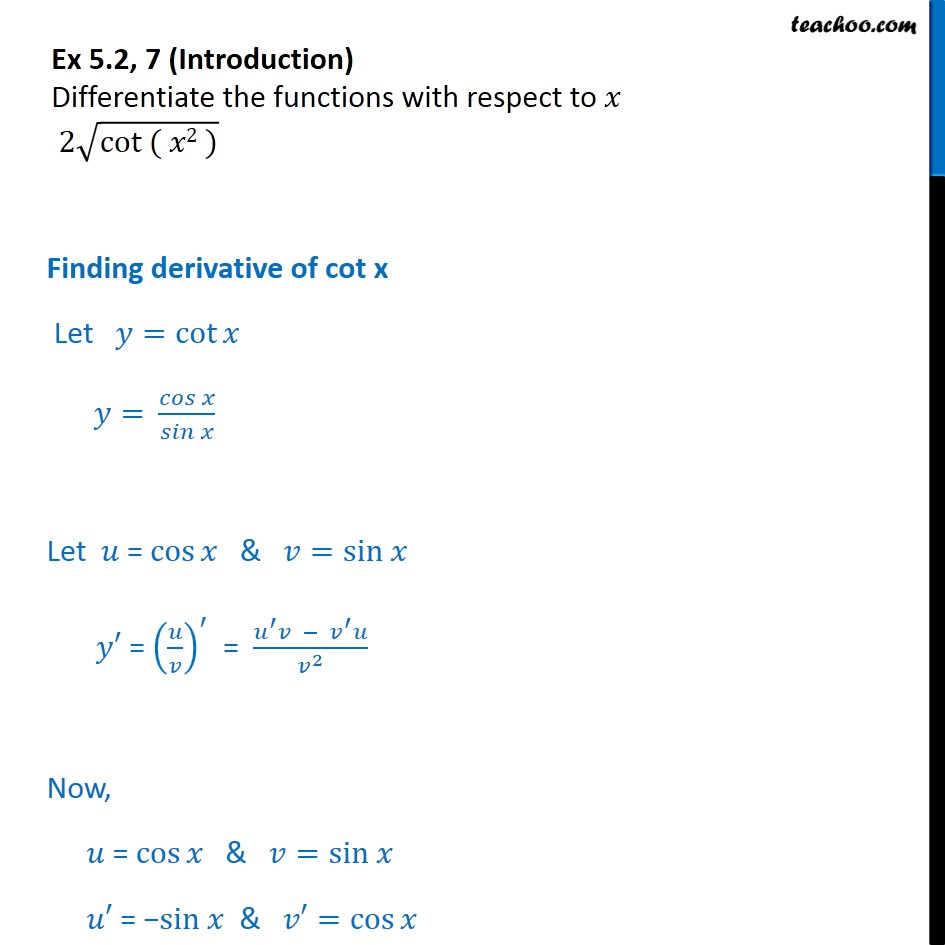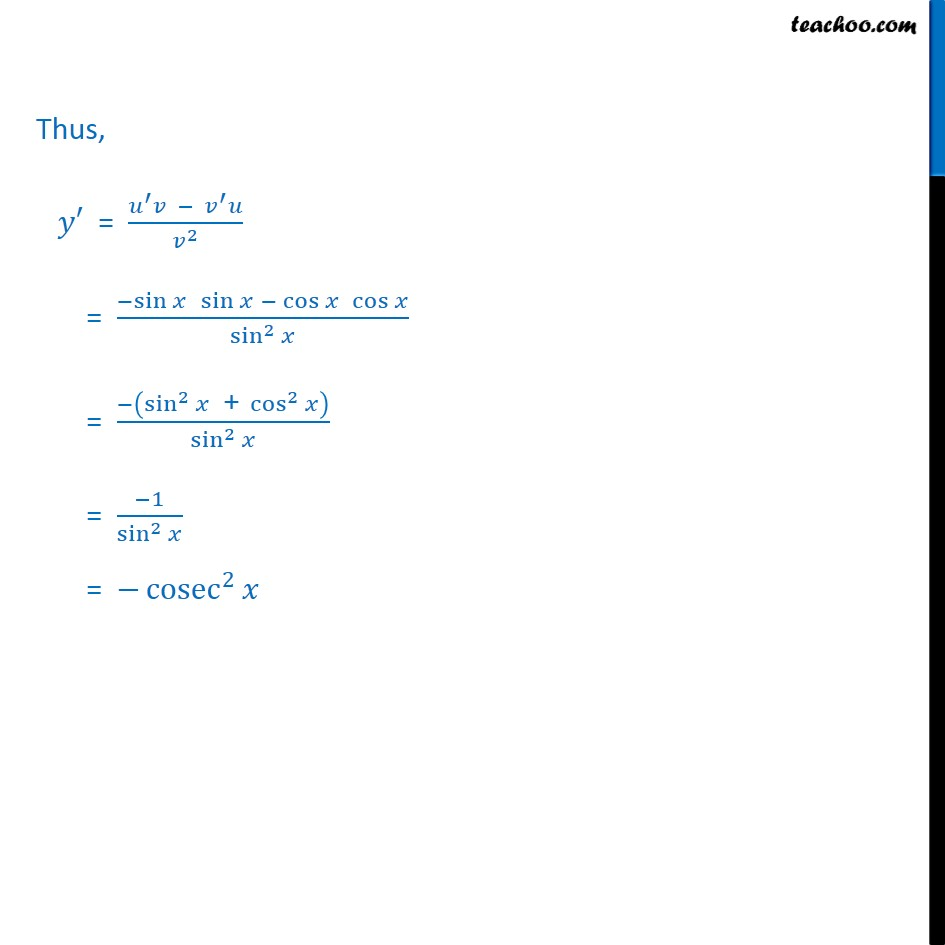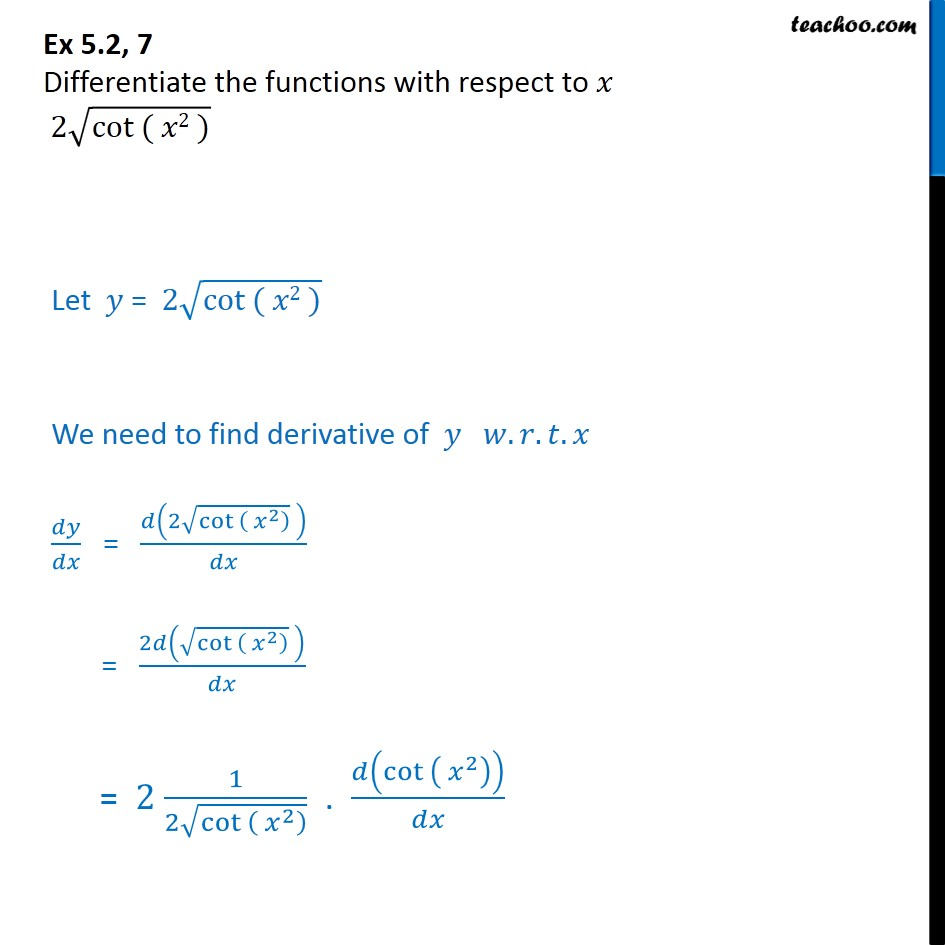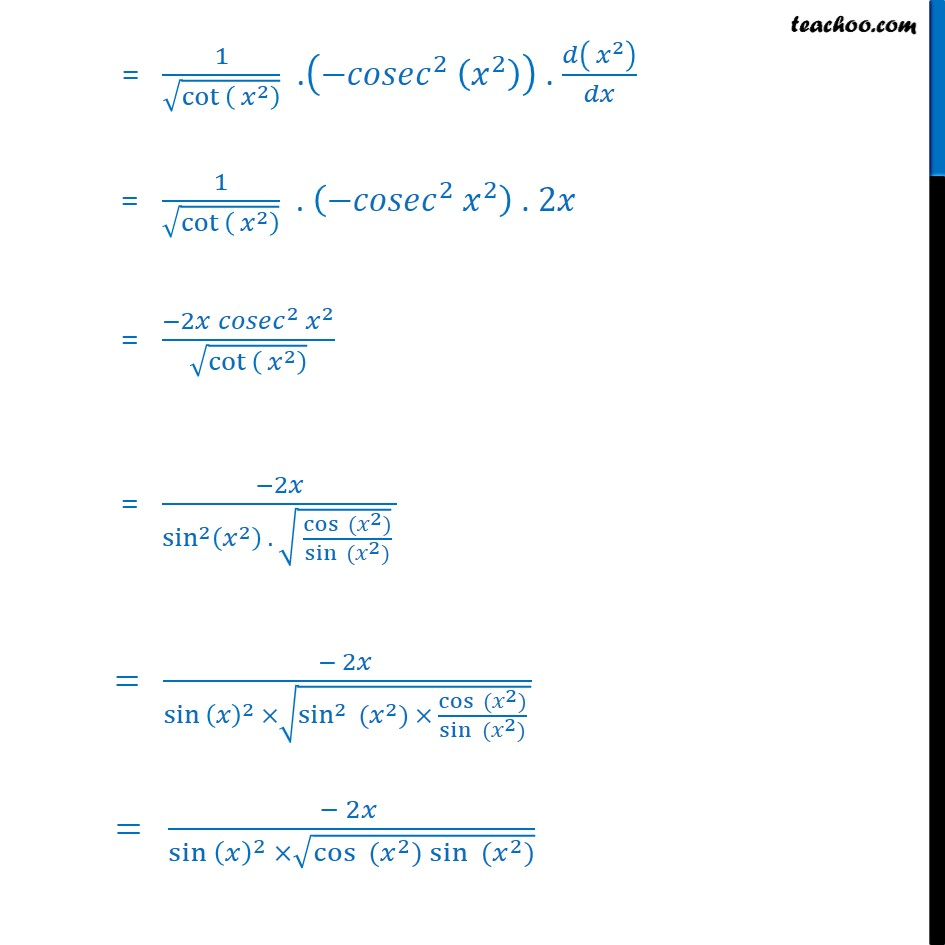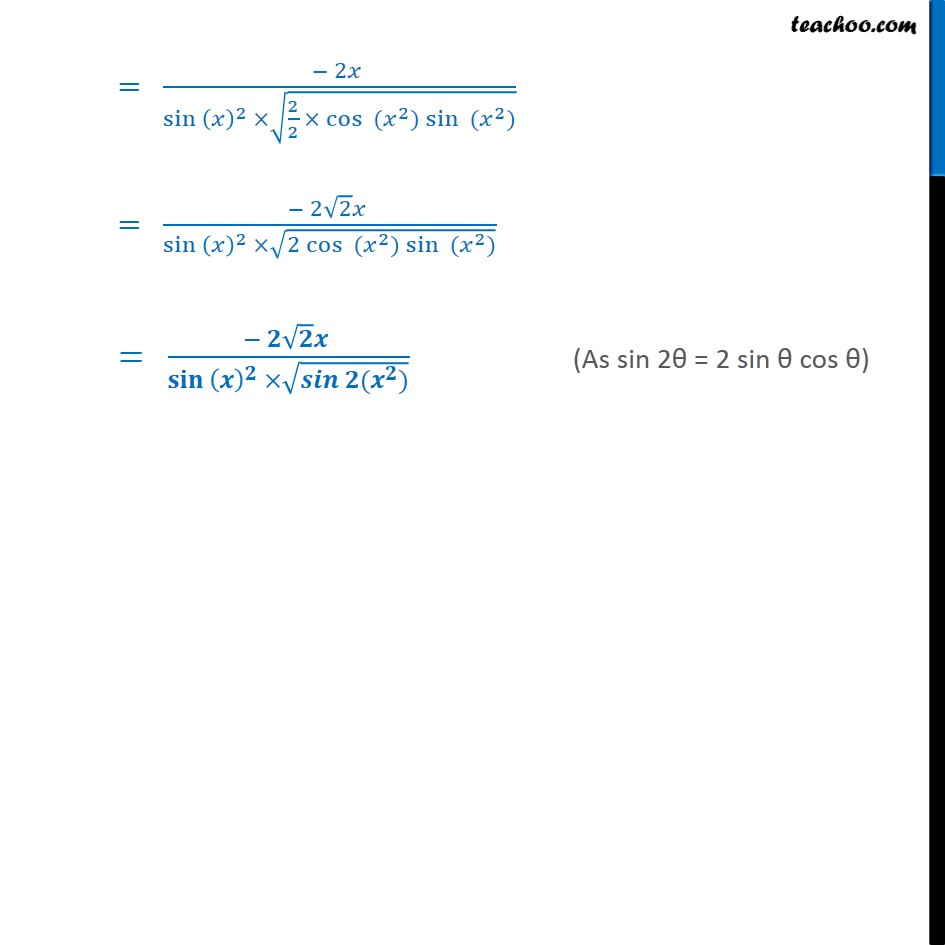1. Chapter 5 Class 12 Continuity and Differentiability
2. Serial order wise
3. Ex 5.2

Transcript

Ex 5.2, 7 (Introduction) Differentiate the functions with respect to 2 (cot ( 2 )) Finding derivative of cot x Let =cot = ( )/( ) Let = cos & =sin = ( / )^ = ( ^ ^ )/ ^2 Now, = cos & =sin = sin & =cos Thus, = ( ^ ^ )/ ^2 = ( sin " " sin " " cos " " cos )/(sin^2 ) = ( (sin^2 " +" cos^2 ))/(sin^2 ) = ( 1)/(sin^2 ) = cosec^2 Thus, = ( ^ ^ )/ ^2 = ( sin " " sin " " cos " " cos )/(sin^2 ) = ( (sin^2 " +" cos^2 ))/(sin^2 ) = ( 1)/(sin^2 ) = cosec^2 Ex 5.2, 7 Differentiate the functions with respect to 2 (cot ( 2 ))

Ex 5.2